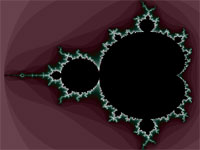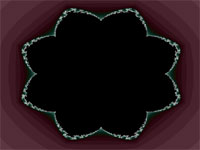### Transformation Formulas

A transformation formula transforms the simple rectangular shape (the default shape coming from the direct mapping of the window to a part of the complex number plane) into another shape. This formula is rather simple to write and understand, because all it does – or better – has to do, is to modify the value of the predefined variable pixel.

A transformation formula can have upto three member function:

1. void init_once(void): Called only once, i.e. when the fractal calculation starts: In this routine you can initialize variables and allocate arrays.
2. void transform(void): This is the main member function: Its purpose is to modify the value of pixel.
3. void description(void): This member function lets you define labels for your parameters, enumeration lists, default values and hints. Each formula can have a description function.

So a transformation mainly consists of a member function void transform(void) which gets called for each pixel before the main fractal loop gets called.

Thus a transformation could look as follows:

`  rotOrder  {  real dAngle;  real dist;       void init_once(void)     {            // nothing to do...     }     void transform(void)     {        dAngle= arg(pixel);        if  (dAngle< 0) {           dAngle=dAngle+2*pi;        }        dist= |pixel|;        dAngle= dAngle-floor(dAngle*4/pi)*pi/4;        if  (dAngle> pi/8) {           dAngle=pi/4-dAngle;        }        pixel= (cos(dAngle)*dist+(0,1)*(sin(dAngle)*dist));     }     void description(void)     {        this.title = "Rotation Symmetry";     }  }  `

Have a look at these images: They show you the effect of that transformation. The transformation applies rotation symmetry to the fractal, so that the area from -22.5 degrees to +22.5 degrees gets repeated 8 times.Standard fractalWith transformation from above.

So all you need to do is to modify the predefined variable pixel!